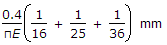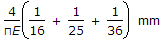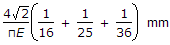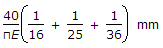# Civil Engineering - UPSC Civil Service Exam Questions

26.

Which of the following are the disadvantages of non-tilting type concrete mixers ?
1. They are not favoured when large sized aggregates are used.
2. Mixing of the concrete occurs through both rolling and pulling from buckets.
3. Content of fines is increased.
4. They are not easy to clean.
Select the correct answer using the codes given below:

 A. 1, 2 and 3 B. 1, 3 and 4 C. 1, 2 and 4 D. 2, 3 and 4

Explanation:

No answer description available for this question. Let us discuss.

27.

A round bar made of same material consists of 3 parts each of 100 mm length having diameters of 40 mm, 50 mm and 60 mm respectively. If the bar is subjected to an axial load of 10 kN, the total elongation of the bar would be (E is the modulus of elasticity in kN/mm2)

 A.B.C.D.Explanation:

No answer description available for this question. Let us discuss.

28.

Which of the following factors have to be considered for the design of the flexible pavement for a highway ?
2. Strength of pavement component material
3. Expansion joints
4. Climatic factors
Select the correct answer using the codes given below :

 A. 1, 2 and 3 B. 2, 3 and 4 C. 1, 3 and 4 D. 1, 2 and 4

Explanation:

No answer description available for this question. Let us discuss.

29.

For practically impervious type of soil, the coefficient of permeability is determined using

 A. variable head test B. constant head test C. consolidation test D. pumping test

Explanation:

No answer description available for this question. Let us discuss.

30.

The basic action involved in sheep foot rolling is

 A. Kneading B. Pressing C. Tamping D. Vibration